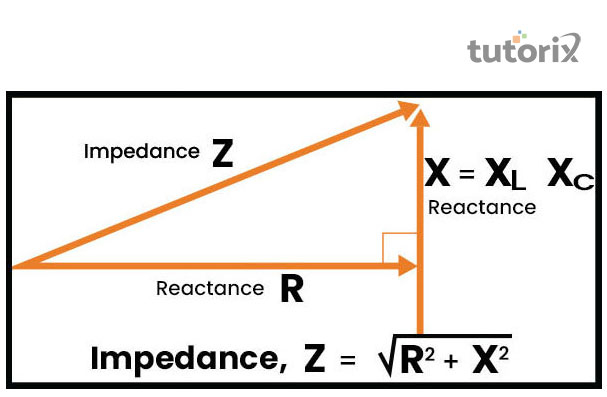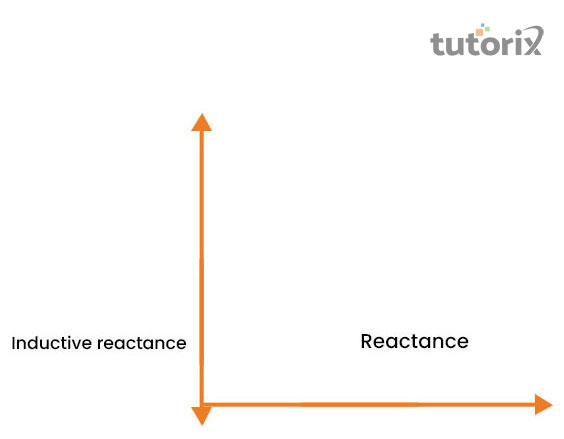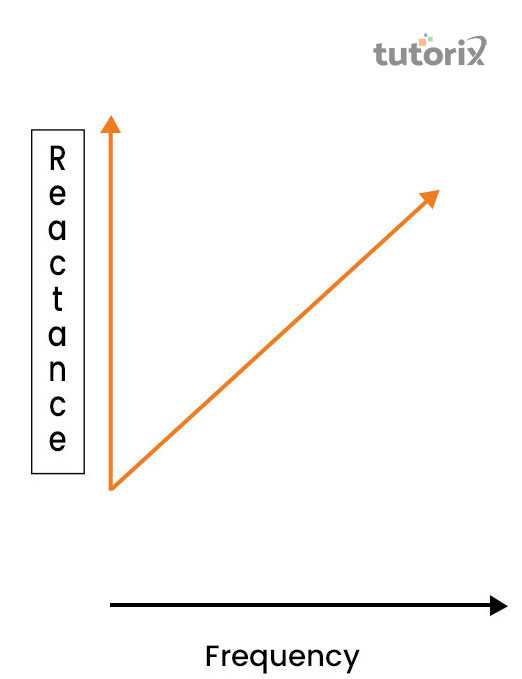# Reactance and Impedance

## Introduction

Reactance refers necessarily to the inertia that opposes the electron’s motions in the circuit of electricity. That means the attribute of the electron’s flow in the electric circuit. Reactance is denoted with the “R” symbol and it is measured in the unit of an ohm (Ω) which is a Greek symbol (Seghir et al. 2018).

Reactance (x) is a combination of Inductive (XL) and Capacitive (Xc).

The formula of Reactance to measure Inductive and Capacitive is X = XL + Xc.

In terms, the phase is presented as “X = R + j X” where X represents reactance and R symbolized the resistance.

## What are Reactance and Impedance?

Reactance is presented in a mathematical ratio with the possible difference of “V” and the flow of current is pointed by “R = VI”. Reactance is also considered the imaginary resistance of a circuit of electrical (Denneman et al. 2020). It refers to all the particles that create obstacles in the electrons flow in the circuit of electricity.Figure 1: Reactance and Impedance

The effective combination of the resistance as well as reactance refers to Impedance. Impedance is a necessary component of electrical circuits to create resistance and reactance as well. “Z” is the mathematical symbol of Impedance it is also measured by ohm (Denneman et al. 2020). It is considered the superset of the combination of resistance and reactance.Figure 2: The components of Impedance

Reactance is the primary component of the opposition that works against the electronic motion. Reactance is mainly presented in electrical as well as magnetic field development. It is mostly seen in the capacitors or inductors (Denneman et al. 2020). Impedance is mainly found in the AC circuit and deals with the entire opposition of the flow of current in terms of resistance and reactance.

## Application of Reactance and Impedance

During the passing alternative current through reactance, a 900 voltage is seen but in terms of impedance, the voltage drops to 00 to 900. Inductive reactance is contributed by the coils and chokes. In the context of the DC circuit, the direction along with the intensity of the electron flow is constant in the electric and magnetic fields (Rao, Prabhu & Mala, 2019).

In the case of Reactance, the flow of electricity is entirely dependent on the wire length and the voltage of electricity.Figure 3: Relation of the frequency with Reactance

In the AC unit, the flow of Reactance is dependent on the change of magnitude as well as the current flow direction. The inductor can be easily passed through the electric circuit at a lower frequency compared to higher frequencies.

## Difference between Reactance and Impedance

ReactanceImpedance
• Reactance is a kind of property of the particles that mainly causes a reduction in the rate of electron flow (Swerdlow et al. 2020).
• No resistors are found in Reactance.
• Reactance depends on the frequency of the Input.
• Impedance refers to the reduction rate, which denotes the electron flow caused by resistance materials or involved in the components (Seghir et al. 2018).
• Impedance comprises a few resistors.
• Impedance already has input frequency,

Table 1: Difference between Reactance and Impedance

## Reactance and Impedance formula

The inductive reactance is defined as “XL = 2πfL” where XL denotes the Inductive reactance that is calculated in ohms, the value of π is around 3.14159, L denotes hertz Inductance, and F refers to hertz Frequency. The formula of Capacitive Reactance is XC = 1/2πFC, where XC refers to capacitive reactance that is measured by ohms and here C denotes farad capacitance (Silva et al. 2020). The formula for circuit impedance is Z = √R2 + X2T. Here Z denotes the impedance, R refers to reactance, and X considers capacitive reactance.

## Conclusion

The current flow, as well as the volume of the voltage, is very dependent on the flow of the electrons that is out of step with each other components. In the case of pure inductive with no resistance, the XL is mainly found to be a representative of the entire opposition f the flow of current. In the value of impedance and inductive reactance is the same then the combination of these two particles generally represents the same magnitude with a minimum of 900 and the angle of the face denotes theta θ. The current phase is expressed always expressed the relative voltage both in leading and lagging. The different elements like resistance, inductance as well as capacitance are mainly seen in the AC circuits. The impedance of the value of these combinations is generally complex in value with the phase or magnitude.

## FAQs

Q1. What are the classifications of Reactance?

Reactance is classified into two different types Capacitive and Inductive reactance. Both reactances is measured by the unit of an ohm.

Q.2. What is the basic comparison between Reactance and Resistance?

Reactance mainly leads to electric power dissipation. Resistance does not lead to any kind of power dissipation.

Q3. What is Wattles's current?

Wattles's current is a type of current that is mainly seen in the AC current that consumes 0 power with more than wattles current. It is calculated with the formula of P = VI cos ϕ.

Q4. What is the application of Reactance?

The application of Reactance is mainly seen in the AC current and the electric circuit. It depends on the flow of electrons. The power of electricity and voltage does not have a great effect on it.

Q5. What is the application of Impedance?

The application of Impedance is seen in AC as well as DC current. It is also found in the electric circuit as well as the magnetic circuits. It denotes a representative of the combination of resistance as well as reactance. Its major application of it as the measurable component of the possible electric circuit can be denoted as the unit of an ohm.

## References

### Journals

Denneman, N., Hessels, L., Broens, B., Gjaltema, J., Stapel, S. N., Stohlmann, J., ... & Oudemans-van Straaten, H. M. (2020). Fluid balance and phase angle as assessed by bioelectrical impedance analysis in critically ill patients: a multicenter prospective cohort study. European Journal of Clinical Nutrition, 74(10), 1410-1419. Retrieved from: https://www.researchgate.net

Rao, H. G., Prabhu, N., & Mala, R. C. (2019). Emulated reactance and resistance by a SSSC incorporating energy storage device. International Journal of Electrical and Computer Engineering, 9(2), 840. Retrieved from: https://research.rug.nl/files/147539522/s41430_020_0622_7.pdf

Seghir, S., Bouthiba, T., Dadda, S., Boukhari, R., & Bouricha, A. (2018). Fault Location in High Voltage Transmission Lines Using Resistance, Reactance and Impedance. Journal of Advanced Engineering and Computation, 2(2), 78-85. Retrieved from: http://jaec.vn/index.php/JAEC/article/viewFile/92/74

Silva, A. M., Nunes, C. L., Matias, C. N., Rocha, P. M., Minderico, C. S., Heymsfield, S. B., ... & Sardinha, L. B. (2020). The usefulness of raw bioelectrical impedance parameters in tracking fluid shifts in judo athletes. European Journal of Sport Science, 20(6), 734-743. Retrieved from: https://www.researchgate.net/profile/Catarina-Nunes-3/publication/335840524_Usefulness_of_raw_bioelectrical_impedance_parameters_in_tracking_fluid_shifts_in_judo_athletes/links/5d9d9be392851c2f70f73495/Usefulness-of-raw-bioelectrical-impedance-parameters-in-tracking-fluid-shifts-in-judo-athletes.pdf

Swerdlow, C. D., Koneru, J. N., Gunderson, B., Kroll, M. W., Ploux, S., & Ellenbogen, K. A. (2020). Impedance in the diagnosis of lead malfunction. Circulation: Arrhythmia and Electrophysiology, 13(2), e008092. Retrieved from: https://www.academia.edu

### Websites

Electronicsclub (2022), reactance and resistance, Available at: https://electronicsclub.info/impedance.htm ) [Accessed on 10th June 2022]